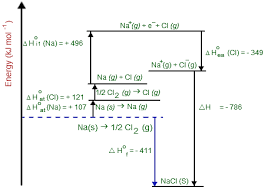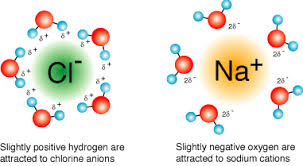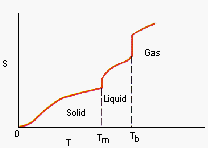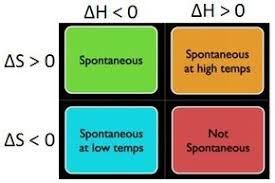# Edexcel Chemistry - Topic 13: Energetics II

?
• Created by: Ryan C-S
• Created on: 12-04-18 21:27

## Enthalpy Changes Definitions

• Enthalpy Change of Formation: The energy transferred when one mole of a compound is formed from its elements at standard conditions
• Enthalpy Change of Atomisation: The enthalpy change when one mol of gaseous atoms are formed from the element in its standard state
• Enthalpy Change of Neutralisation: The enthalpy change when solutions of acid and alkali react together to form one mole of water under standard conditions
• First Ionisation Enthalpy: The enthalpy change required to remove one mole of electrons from one mole of gaseous atoms to form one mole of gaseous 1+ ions
• Second Ionisation Enthalpy: The enthalpy change required to remove one mole of electrons from one mole of gaseous 1+ ions to form one mole of gaseous 2+ ions
• First Electron Affinity: The enthalpy change that occurs when one mole of gaseous atoms gain one mole of electrons to form one mole of gaseous 1- ions
• Second Electron Affinity: The enthalpy change that occurs when one mole of gaseous 1- ions gain one mole of electrons to form one mole of gaseous 2- ions
• Ethalpy of Latice Formation: The standard enthalpy change when one mole of an ionic crystal lattice is formed from its constituent ions in gaseous forms
1 of 12

## Lattice Enthalpy

• The enthalpy of lattice formation is the standard enthalpy change when one mole of an ionic crystal lattice is formed from its constituent ions in gaseous form
e.g. Na+(g) + Cl-(g) --> NaCl(s)
• The strength of an ethalpy of lattice formation depends on:

The Size of Ions

• The larger the ions, the less negative the enthalpies of lattice formation and the weaker a lattice
• Larger ions cause the charges to be further apart and so the weaker the attraction between the ions

The Charges of Ions

• The bigger the charge of the ion, the greater the attractive force between the ions so the stronger the lattice enthalpy (the more negative the enthalpy)
2 of 12

## Born Haber Cycles

• Born-Haber Cycles can be used to determine lattice enthalpies which cannot be determined directly
• Hess's Law can be applied as the enthalpy of formation will equal the sum of all the other enthalpies involved3 of 12

## Ionic Model and Polarisation

• Theoretical lattice enthalpies assume a perfect ionic model when the ions are 100% ionic and spherical and the attractions are purely electrostatic
• The Born-Haber lattice enthalpy is the real experimental value.
• Polarisation is caused by the distortion of a negative ion by a positive ion
• When 100% ionic, the ions are spherical and the Born-Haber lattice enthalpy and theoretical values are the same
• When a polarising effect is placed on an anion, it can distort the charge cloud but the Born-Haber lattice enthalpy and theoretical values differ

The Polarising Power of a cation increases when:

• The positive ion has a smaller ionic radius
• The positive ion has a larger charge

The Polarisability of the anion depends on its size; the bigger the ion, the more easily it is distorted

4 of 12

## Trends in Lattice Enthalpies

Size of the Ions

• The larger the ionic radius, the further the charges are between each other and the weaker the attractive force between the ions so the less negative the enthalpies of lattice formation

Charge of the Ions

• The bigger the charge, the greater the positive charge of the cation and so the stronger the attractive force between the ions so the more negative the enthalpies of lattice formation
• Lattice enthalpies become less negative down a group as the ionic radius increases and there is more shielding
• Lattice enthalpies become more negative across a period of metals as the ion becomes more positive increasing the nuclear attractive force
5 of 12

## Enthalpies of Hydration and Solutions

• Enthalpy of Hydration: The enthalpy change when one mole of gaseous ions becomes hydrated such that further dilution causes no further heat change
X+(g) + aq --> X+(aq)
• Enthalpy of Solutions: The standard enthalpy change when one mole of an ionic solid dissolves in a large enough volume of water to ensure that dissolved ions are well seperated and don't interact with one another
NaCl(s) + aq --> Na+(aq) + Cl-(aq)

When an ionic lattice dissolves in water, it involves breaking up the bonds and forming new bonds between the ions and water molecules.
Hydration enthalpies are Exothermic as energy is given out as water molecules bond to metal ions

6 of 12

## Solubility

• When an ionic compound is dissolved in water, the negative ions are attracted to the δ+ hydrogen atoms and the positive ions are attracted to the δ- oxygen atom
• If a substance is insoluble it is often because the lattice enthalpy is much larger than the hydration enthalpy and it isn't energetically favourable to break up. Therefore the enthalpy of solutions is endothermic
• When a solid dissolves into ions, entropy increases as there is more disorder as the solid changes to liquid solutions and the number of particles increases7 of 12

## Entropy

• Entropy is a description of the number of ways atoms can share quanta of energy. If there is a high number of ways atoms can share energy (W) then the system is disordered and entropy (S) is high
• Substances with more ways of arranging their atoms and energy (more disordered) have higher entropies
• Solids have lower entropies than liquids or gases as there are fewer ways to organise the atoms and energies
• Gases have the largest entropies as they are much more disordered and have the largest energies8 of 12

## Calculating Entropy

∆S˚system = Σ S˚products - ΣS˚reactants

∆S˚surroundings = -∆Hreaction / Temperature

∆S˚total = ∆S˚system + ∆S˚surrounding

The Unit of Entropy is: J K-1 mol-1

• In an exothermic reaction, energy is given out to the surroundings. The number of ways of arranging the energy increases and so ∆S˚surroundings increases and is positive
• In an endothermic reaction, energy is taken in from the surroundings. The number of ways of arranging the energy decreases and so ∆S˚surroundings decreases and is negative
• For any reaction to be spontaneous∆S˚total must be positive. If the reaction is not spontaneous (doesn't go), the ∆S˚total will be negative
9 of 12

## Gibbs Free Energy Change

• Gibbs Free Energy Change is a term that combines the effects of entropy and enthalpy into one number
• ∆G = ∆H - T∆S˚system
• For any spontaneous change, ∆G will be Negative
• A reaction that has increasing entropy (+ve ∆S˚system) and is exothermic (-ve ∆H) will make ∆G be negative and will always be feasible
• If ∆G is negative, there may be a chance a reaction will not be feasible because of a high activation energy or another kinetic reason
• As physical phase changes (e.g. Melting and Boiling Points) are equilibria ∆G will be zero10 of 12

## Effects of Temperature on Gibbs Free Energy

• Changing temperature will change the value of -T∆Ssystem

If the reaction involves an increase in entropy (∆Ssystem is +ve) then increasing temperature will make it more likely that ∆G is negative and more likely that a reaction will occur

If the reaction involves a decrease in entropy (∆Ssystem is -ve) then increasing temperature will make it less likely that ∆G is negative and less likely that a reaction will occur

If the reaction has a ∆Ssystem that is close to zero then increasing temperature will not have a large effect on the feasibility of a reaction as -T∆Ssystem will be small and G will not change much

11 of 12

## Determining the Feasibility of a Reaction

Total Entropy (∆Stotal)
If ∆Stotal is positive a reaction is feasible

Gibbs Free Energy (∆G)
If ∆G is negative a reaction is feasible

Electrode Potentials (E˚)
If E˚ is positive a reaction is feasible (see Topic 14)

Equilibrium Constant (Kc)
If Kc < 10^-10 a reaction isn't feasible
If Kc ~ 0.1 the reaction is in equilibrium favouring the Reactants
If Kc = 1 the reaction is in equilibrium producing equal amounts of Reactants and Products
If Kc ~ 10 the reaction is in equilibrium favouring the Products
If Kc > 10^10 the reaction goes to completion

12 of 12